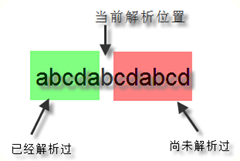### 正则表达式之反向引用和前瞻后顾

``````\$num = "123456789";
\$num = preg_replace('/(?<=[0-9])(?=(?:[0-9]{3})+(?![0-9]))/', ',', \$num);
echo \$num;
``````

### 反向引用

``````preg_match('/^\d{4}([\-\_])\d{2}\1\d{2}\s\d{2}(\:)\d{2}\2\d{2}\$/', \$str)；
``````

### 正则的方向### 前瞻后顾

``````\$str = "garen.goh and ugood are good names."
``````

``preg_replace('/(?<=\s)g(?=ood\s)/', 'G', \$str);``

``preg_replace('/(?<!\s)g|g(?!ood\s)/', 'G', \$str);``

Java对后顾功能的支持度，可以用?符号了，不能用+号，因为+号实在太扯淡，能匹配一个字符，也能匹配一万个字符，人家Sun公司的码农根本实现不出来，所以不给你提供这个功能。

.NET对后顾功能的支持度最高，可以匹配可变长度字符，可以用+号了，不愧是世界首富比尔盖茨微软，多砸钱，不信有实现不出来的功能。

### 深入理解

``````\$num = preg_replace('/(?<=[0-9])(?=(?:[0-9]{3})+(?![0-9]))/', ',', \$num);
``````

``````preg_replace('/(?<=\d)(?=(\d{3})+(?=\.))/', ',', \$str);	123456789==>123456789
``````

``````preg_replace('/(?<=\d)(?=(\d{3})+(?=\.|\$))/', ',', \$str);	123456789.987654321==>123,456,789.987,654,321
``````

``````preg_replace('/(?<=\d)(?=(\d{3})+(?=\.)|(?<!\.\d*)(\d{3})+(?=\$))/', ',', \$str);
``````